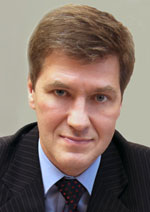Publications
Editor-in-ChiefNikiforov
D.Sc., Prof.
Partners

# METHODS OF TEMPERATURE FIELD MODELING FOR CONTACTLESS LASER DEFORMATION OF A PLATE

S. Lukyanenko, I. Mykhailova

Read the full article';

Abstract

Contactless laser deformation is a process of changing a geometrical form of an object by its heating and cooling without using mechanical forces. One of the factors, influencing deformation, is heating, which creates temperature field and overpatching speed of temperature field.
The article deals with a computer modeling result for temperature field of a plate irradiated by a moving laser beam. Comparison of results, obtained by using two mathematical models and experiments, is conducted. Such parameters as density, thermal capacity and thermal conductivity are constant values in the first model, and they are linearly dependent on temperature in the second one. Linear approximation of table values by least-squares method is used to define this dependence. Both models have the temperature field computed by 3D non-stationary heat equation: it is linear function in first model and quasilinear function in the second. To solve the equation a six- step implicit finite difference coordinate-wise splitting scheme is applied, which has the second order of accuracy for all coordinates. Systems of linear algebraic equations, created by this finite difference scheme, are solved by modified Gauss method. Adaptive method is used for automatic design of variable difference mesh. It condenses nodes in high gradient zones and dilutes them in regions where temperature changes gradually. This gives the possibility for computation time shortening and receiving a result with predefined accuracy. Results of computer modeling show that taking into account dependence of material parameters on temperature produces more accurate results. However, it requires more steps and therefore it is more time-consuming.

Keywords: 3D non-stationary quasi-linear heat equation, difference approximation method

References
1. Price S. Laser forming // Journal of Manufacturing Science and Engineering. 2007. V. 129. P. 117–124.
2. Shi Y., Shen H., Yao Z., Hu J. Temperature gradient mechanism in laser forming of thin plates // Optics & Laser Technology. 2007. V. 39. N 4. P. 858–863.
3. Головко Л.Ф., Лук’яненко С.О., Смаковський Д.С., Михайлова І.Ю., Агеєнко В.А. Моделювання температурного поля при зміцненні матеріалів лазерним випромінюванням // Моделювання та інформаційні технології. 2008. № 45. С. 28–35.
4. Кутателадзе С.С. Основы теории теплообмена. М.: Атомиздат, 1979. 416 с.
5. Калиткин Н.Н. Численные методы. М.: Наука, 1978. 512 с.
6. Лук’яненко С.О. Адаптивні обчислювальні методи моделювання об’єктів з розподіленими парамет- рами. Київ: Політехніка, 2004. 236 с.
7. Марчук Г.И. Методы вычислительной математики. М.: Наука, 1977. 456 с.
8. Тихонов А.Н., Самарский А.А. Уравнения математической физики. M.: Наука, 1977. 735 с.
9. 65Г – сталь конструкционная рессорно-пружинная. Марочник стали и сплавов [Электронный ресурс]. Режим доступа: http://www.splav.kharkov.com/mat_start.php?name_id=265, свободный. Яз. рус. (дата обращения 07.11.2013).
10. Михайлова І.Ю. Спосіб функціонального тестування точності результатів, отриманих з використан- ням адаптивної сітки // Математичне та комп’ютерне моделювання. Сер. Технічні науки. 2012. В. 7. С. 124–132.